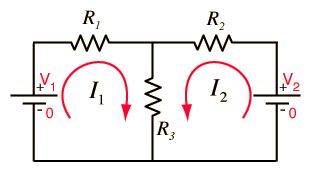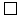# DC Circuit Examples

The basic tools for solving DC circuit problems are Ohm's Law, the power relationship, the voltage law, and the current law. The following configurations are typical; details may be examined by clicking on the diagram for the desired circuit.Index

DC Circuits

 HyperPhysics***** Electricity and Magnetism R Nave
Go Back

# Two Loop Circuits

A circuit with two loops and two sources is involved enough to illustrate circuit analysis techniques. It may be analyzed by direct application of the voltage law and the current law, but some other approaches are also useful.Given the voltages, current analysis may be carried out by:Voltage and current lawsSuperposition theoremThevenin's theoremNorton's theorem
 Other DC circuit examples
Index

DC Circuits

 HyperPhysics***** Electricity and Magnetism R Nave
Go Back